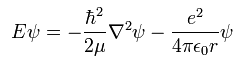## Wave Equation for the Hydrogen Atom

#### Learning Objective

• Explain how the solution of the Schrödinger equation for the hydrogen atom yields the four quantum numbers and use these to identify degenerate states

#### Key Points

• The Schrödinger equation is a differential equation that provides a way to calculate the possible wavefunctions of a system and describes how they dynamically change in time.
• Particles can behave like waves because their corresponding wavefunction satisfies the Schrödinger equation.
• The motion of a particle, described by a wave packet solution to the Schrödinger equation, is also described by the Hamilton–Jacobi equation of motion.

#### Terms

• Hamiltonian

In quantum mechanics, the observable, denoted by H, that corresponds to the total energy of the system.

• eigenfunctions

A class of mathematical function that is non-zero and returns the same function except for a multiplicative scaling factor when acted on by a linear operator (such as a Hamiltonian).

• quantum number

One of certain integers or half-integers that specify the state of a quantum mechanical system (such as an electron in an atom).

The solution of the Schrödinger equation for the hydrogen atom uses the fact that the Coulomb potential produced by the nucleus is isotropic—it is radially symmetric in space and only depends on the distance to the nucleus. Although the resulting energy eigenfunctions (the orbitals) are not necessarily isotropic themselves, their dependence on the angular coordinates follows generally from this isotropy of the underlying potential. The eigenstates of the Hamiltonian (that is, the energy eigenstates) can be chosen as simultaneous eigenstates of the angular momentum operator. This corresponds to the fact that angular momentum is conserved in the orbital motion of the electron around the nucleus. Therefore, the energy eigenstates may be classified by two angular momentum quantum numbers, ℓ and m(both are integers). The angular momentum quantum number ℓ = 0, 1, 2, … determines the magnitude of the angular momentum. The magnetic quantum number m= −, …, +ℓ determines the projection of the angular momentum on the (arbitrarily chosen) z-axis and therefore the orientation of the orbital in three-dimensional space.

In addition to mathematical expressions for total angular momentum and angular momentum projection of wavefunctions, an expression for the radial dependence of the wavefunctions must be found. It is only here that the details of the 1/r Coulomb potential enter (leading to Laguerre polynomials in r). This leads to a third quantum number, the principal quantum number n = 1, 2, 3, …. The principal quantum number in hydrogen is related to the atom’s total energy. Note that the maximum value of the angular momentum quantum number is limited by the principal quantum number: it can run only up to n − 1, i.e. ℓ = 0, 1, …, n − 1.

## Degeneracy of Different Magnetic Quantum Numbers

Due to angular momentum conservation, states of the same ℓ but different mhave the same energy. This holds for all problems with rotational symmetry. For the hydrogen atom, states of the same n but different ℓ are also degenerate (they have the same energy). This is a specific property of hydrogen and is not true for more complicated atoms. These atoms have an effective potential differing from the 1/r form due to the presence of the inner electrons shielding the nucleus potential.

The spin of the electron adds the last quantum number, the projection of the electron’s spin angular momentum along the z-axis, which can take on two values. Therefore, any eigenstate of the electron in the hydrogen atom is described fully by four quantum numbers. According to the usual rules of quantum mechanics, the actual state of the electron may be any superposition of these states. This explains also why the choice of z-axis for the directional quantization of the angular momentum vector is immaterial: an orbital of given ℓ and m′ obtained for another preferred axis, z′,can always be represented as a suitable superposition of the various states of different m (but same ℓ) that have been obtained for z.

Using a three-dimensional approach, the following form of the Schrödinger equation can be used to describe the hydrogen atom:Schroedinger Equation Three dimensional Schrödinger equation as applied to the H atom.

where $\mu$ is the reduced mass of the electron-proton pair. The wavefunction itself is expressed in spherical polar coordinates:

$\psi \left( r,\theta ,\phi \right) =R\left( r \right) { Y }_{ l }^{ m }\left( \theta ,\phi \right) =R\left( r \right) \Theta \left( \theta \right) \Phi \left( \phi \right)$

where R are radial functions and theta ($\theta$) and phi ($\phi$) are spherical harmonic terms. The generalized family of solutions is:

${\psi }_{ n,l,m }\left( r,\theta ,\phi \right) =\sqrt { { \left( \frac { 2 }{ n{ a }_{ 0 } } \right) }^{ 3 }\frac { \left( n-l-1 \right) ! }{ { 2n[(n+l)! ] }^{ 3 } } } { e }^{ { -r }/{ { na }_{ 0 } } }{ (\frac { 2r }{ n{ a }_{ 0 } } ) }^{ l }{ L }_{ n-l-1 }^{ 2l+1 }(\frac { 2r }{ n{ a }_{ 0 } } )\cdot { Y }_{ l }^{ m }(\theta ,\phi )$

where a0 is the Bohr radius, L are the generalized Laguerre polynomials, and n, l, and m are the principal, azimuthal, and magnetic quantum numbers, respectively.

#### Sources

Boundless vets and curates high-quality, openly licensed content from around the Internet. This particular resource used the following sources:

“Boundless.”

http://www.boundless.com/
Boundless Learning
CC BY-SA 3.0.

“quantum number.”
“Hamiltonian.”
“Schr&#246;dinger equation.”